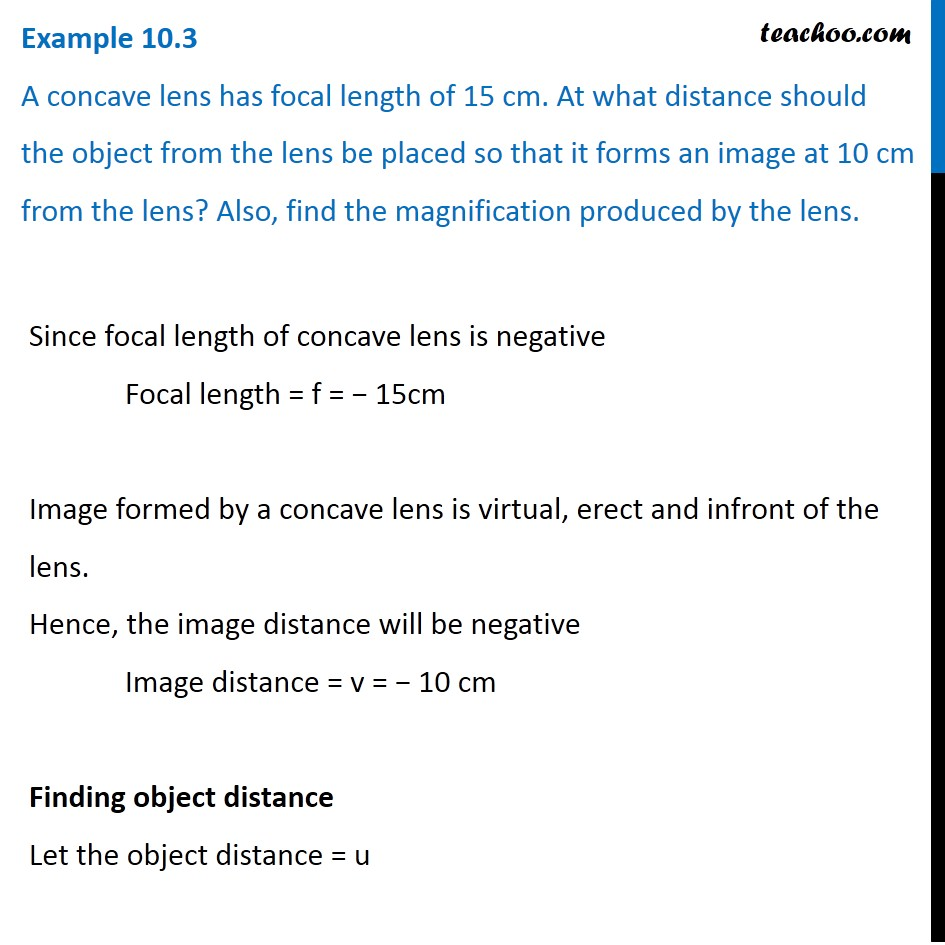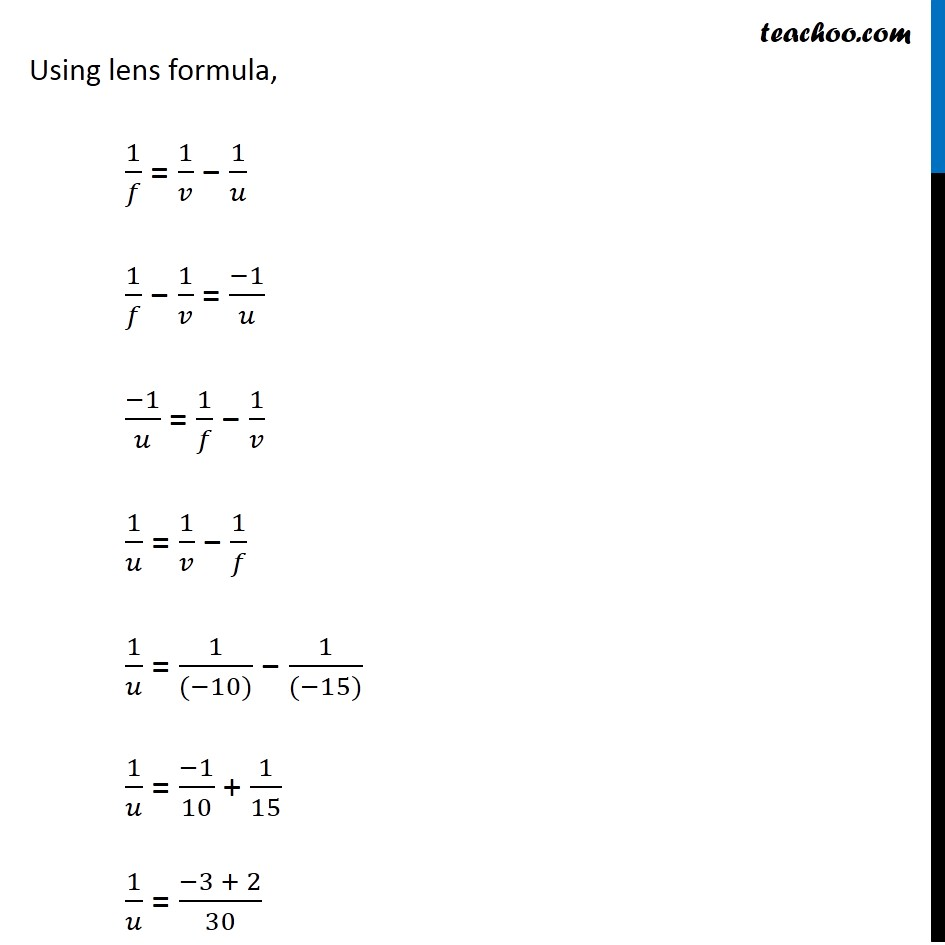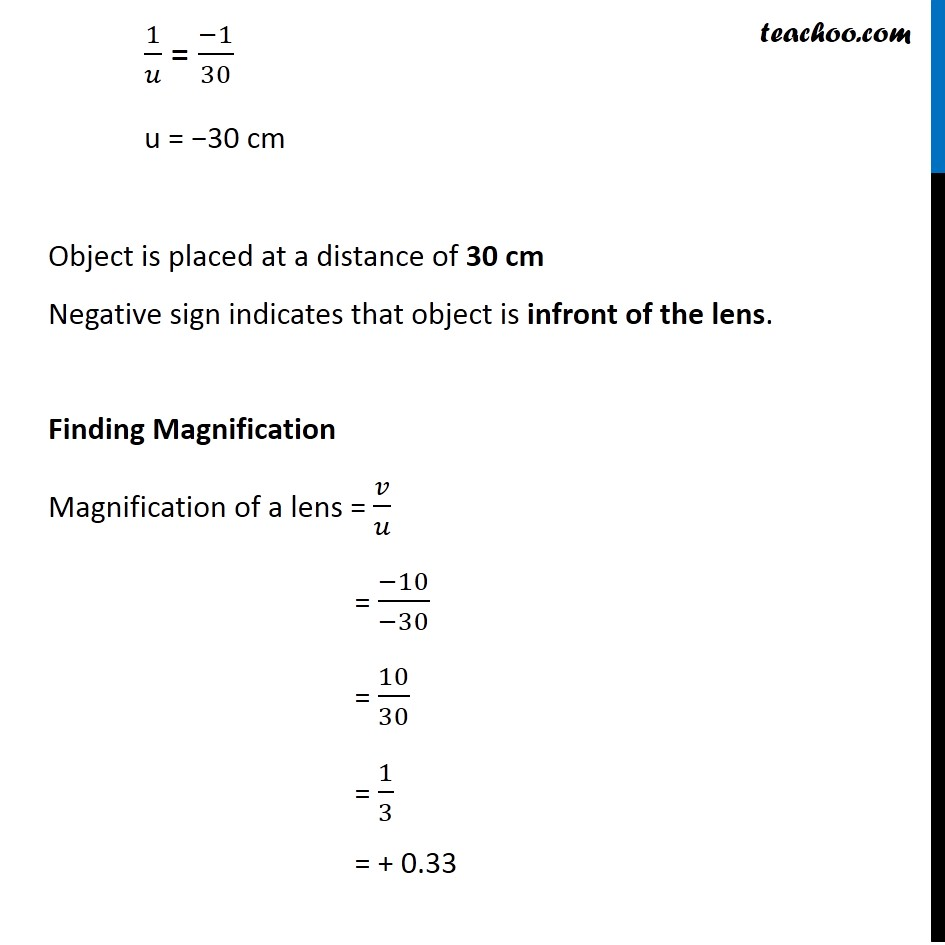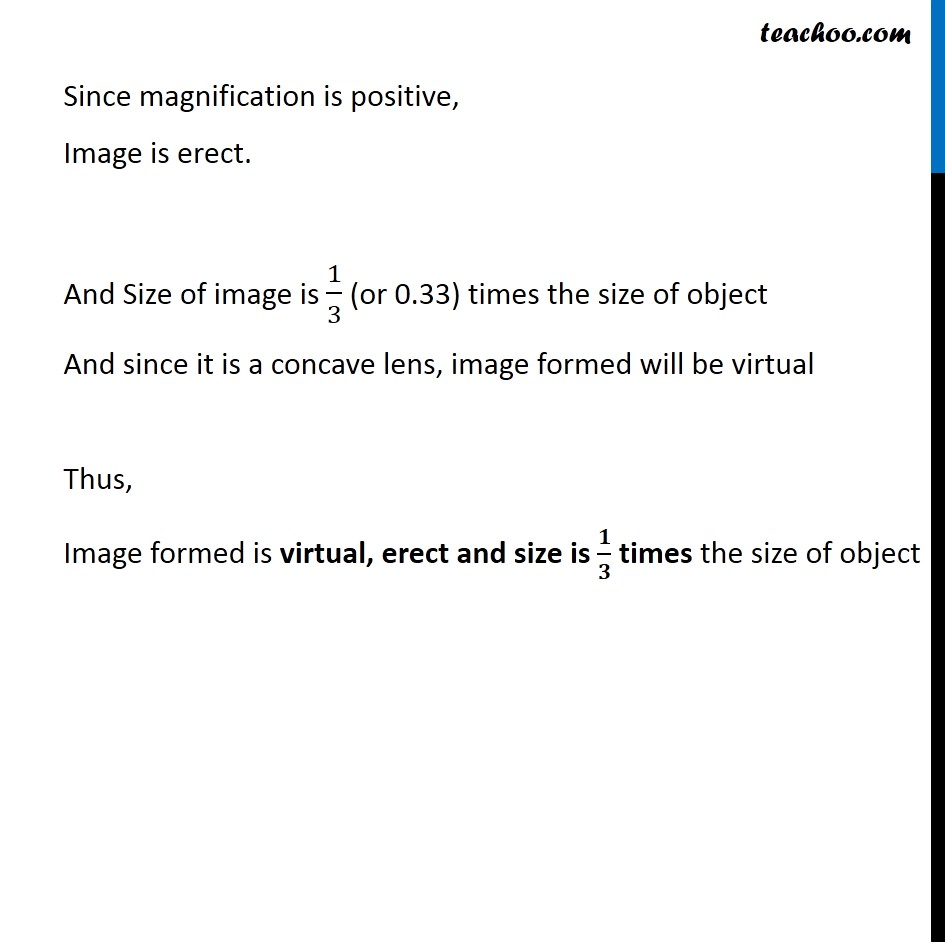Examples from NCERT Book

Class 10
Chapter 10 Class 10 - Light - Reflection and Refraction

## A concave lens has focal length of 15 cm. At what distance should the object from the lens be placed so that it forms an image at 10 cm from the lens? Also, find the magnification produced by the lens.Learn in your speed, with individual attention - Teachoo Maths 1-on-1 Class

### Transcript

Example 10.3 A concave lens has focal length of 15 cm. At what distance should the object from the lens be placed so that it forms an image at 10 cm from the lens? Also, find the magnification produced by the lens. Since focal length of concave lens is negative Focal length = f = − 15cm Image formed by a concave lens is virtual, erect and infront of the lens. Hence, the image distance will be negative Image distance = v = − 10 cm Finding object distance Let the object distance = u Using lens formula, 1/𝑓 = 1/𝑣 − 1/𝑢 1/𝑓 − 1/𝑣 = (−1)/𝑢 (−1)/𝑢 = 1/𝑓 − 1/𝑣 1/𝑢 = 1/𝑣 − 1/𝑓 1/𝑢 = 1/((−10)) − 1/((−15)) 1/𝑢 = (−1)/10 + 1/15 1/𝑢 = (−3 + 2)/30 1/𝑢 = (−1)/30 u = −30 cm Object is placed at a distance of 30 cm Negative sign indicates that object is infront of the lens. Finding Magnification Magnification of a lens = 𝑣/𝑢 = (−10)/(−30) = 10/30 = 1/3 = + 0.33 Since magnification is positive, Image is erect. And Size of image is 1/3 (or 0.33) times the size of object And since it is a concave lens, image formed will be virtual Thus, Image formed is virtual, erect and size is 𝟏/𝟑 times the size of object